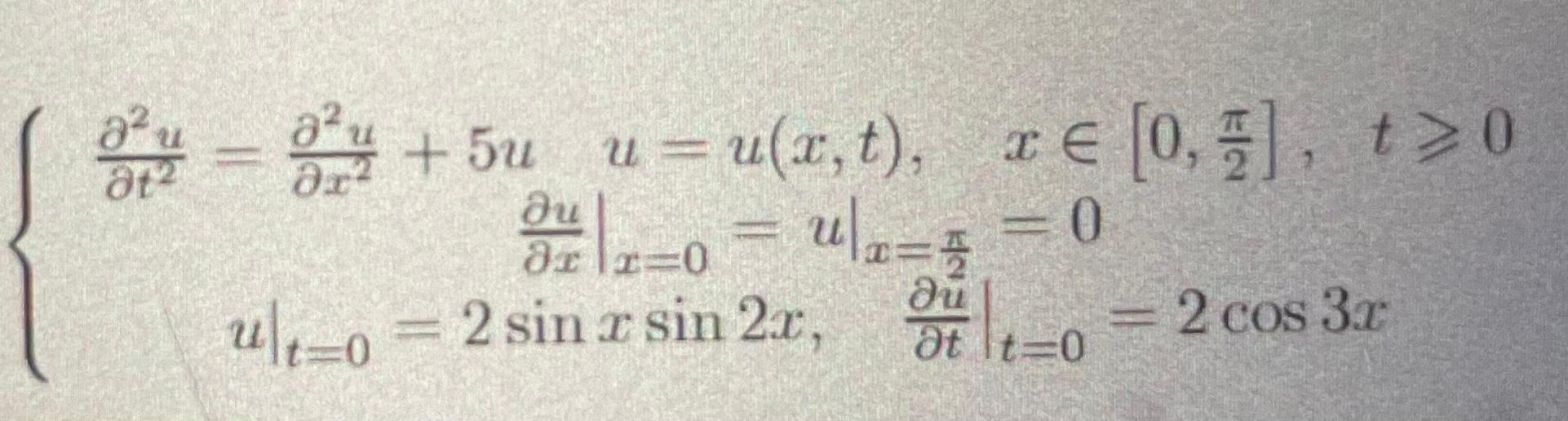Home / Expert Answers / Other Math / t22u-x22u-5uu-u-x-t-x-0-2-t0xux-0-pa428

# (Solved): t22u=x22u+5uu=u(x,t),x[0,2],t0xux=0= ...We have an Answer from Expert

As mentioned before, the given equation is a partial differential equation known as the nonlinear sine-Gordon equation. Here, we'll solve this PDE numerically using the finite difference method, which involves discretizing the spatial and temporal domains.

Let's begin by defining the necessary parameters and initial/boundary conditions:

- Let N be the number of grid points in the x direction
- Let M be the number of time steps
- Let ?x be the spacing between the grid points in the x direction
- Let ?t be the time step
- Define the initial condition as u[i, 0] = 2*sin(i*?x)*sin(2*i*?x)
- Define the boundary conditions as u[0, j] = u[N-1, j] = 0

Now, we can implement the finite difference method to compute the numerical solution. At each time step, we'll iterate over the interior grid points (excluding the boundary points) and use the following numerical scheme to compute the solution at the next time step:

Note that this scheme involves computing the second derivatives of u using finite differences and using the values at the current and previous time steps to compute the next time step.

Here's the Python code to implement the finite difference method:

`
import numpy as np
import matplotlib.pyplot as plt

# Define parameters and initial/boundary conditions
# Number of grid points
# Number of time steps
# Length of domain
We have an Answer from Expert